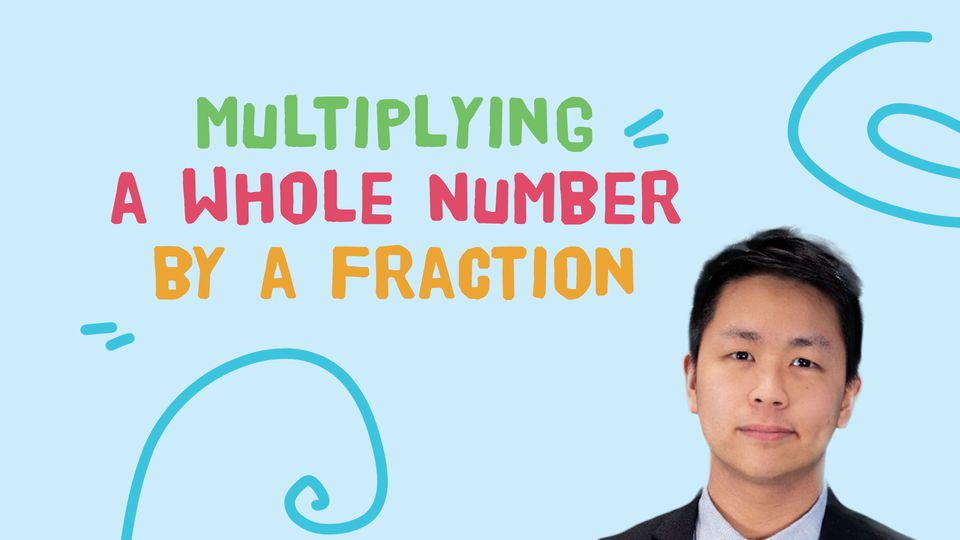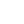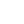Preview the Course
55m

# Multiplication - Multiplying a Whole Number by a Fraction

by Ivan I. | Math FREE9 Lessons 55:06
1.1. Introduction 1 2:41
2.2. Do Now 2:31
3.3. Do Now Answers Part 1 7:55
4.4. Do Now Answers Part 2 8:14
5.5. Steps 7:09
6.6. Practice 4:49
7.7. Word Problems 8:41
8.8. Worksheet 2:36
9.9. Worksheet Answers 10:30

55m

NGSS Standards

FREE

Course

Fractions, specifically multiplying fractions, is important for use in many areas of everyday life. If I want to triple a recipe that calls for ⅓ cup of sugar, I need to be able to multiply a whole number times a fraction to know how much sugar to add. If I have a shirt that's on sale for ½ of its original price of \$19, I need to multiply a fraction by a whole to get my answer. In this class, we will learn about how we can multiply whole numbers with fractions. We will discover how we can solve word problems and show our work with different real-life examples.

Tags:

• Elementary School
• Fractions
• Learning
• Math
• Multiply
• Whole Numbers

## Instructor

I have tutored hundreds of students in various subjects, formats, and locations. I have taught students both online and in-person subjects ranging from chess, SAT, ACT, computer science, SHSAT, and personal finance. I have hosted large classes as well as one-on-one sessions. In high school, I have taught SHSAT at a tutoring school, chess at a non-profit organization and for an after school program. As a college student, I host online chess workshops as well as private lessons. I cater my lessons to the needs of the class and individual and strive to make the topic engaging, interactive, and fun!

See full profile